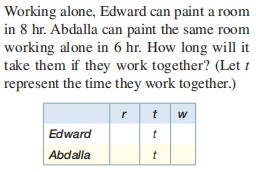### Still have math questions?

Algebra
QuestionWorking alone, Edward can paint a room in $$8 hr$$ . Abdalla can paint the same room working alone in $$6 hr$$ . How long will it take them if they work together? (Let $$t$$ represent the time they work together.)

$$\frac { 1 } { 8 } t + \frac { 1 } { 6 } t = 1 , \text { or } \frac { 1 } { 8 } + \frac { 1 } { 6 } = \frac { 1 } { t }$$